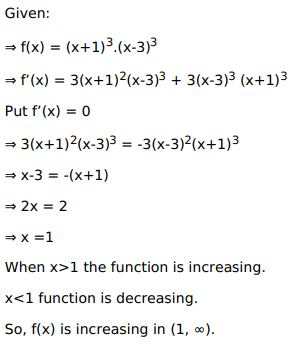# Solve this following

Question:

Mark $(\sqrt{)}$ against the correct answer in the following:

$f(x)=(x+1)^{3}(x-3)^{3}$ is increasing in

A. $(-\infty, 1)$

B. $(-1,3)$

C. $(3, \infty)$

D. $(1, \infty)$

Solution: